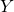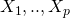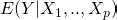# Why shouldn’t I use linear regression if my outcome is binary?

This week a student asked me (quite reasonably) whether using linear regression to model binary outcomes was really such a bad idea, and if it was, why. In this post I’ll look at some of the issues involved and try to answer the question.

Linear regression assumptions
The linear regression model is based on an assumption that the outcomeis continuous, with errors (after removing systematic variation in mean due to covariates) which are normally distributed. If the outcome variable is binary this assumption is clearly violated, and so in general we might expect our inferences to be invalid.

Actually things might not (necessarily) be too bad. The assumption of conditional normality will obviously not hold if the outcome is binary. But if the assumed form for how the expectation of the outcome depends on the covariates is correct, i.e.is correct, the linear regression parameter estimates are unbiased. However, our standard errors and therefore confidence intervals, which are by default calculated assuming normality for the outcome (conditional on covariates) will be invalid.

The conditional variance is not constant
With binary data the variance is a function of the mean, and in particular is not constant as the mean changes. This violates one of the standard linear regression assumptions that the variance of the residual errors is constant. However, we can mitigate this by using robust sandwich standard errors.

The normality assumption
The usual inference procedures for linear regression assume that the residual errors are normally distributed. However, provided the sample size is not small, thanks to the central limit theorem confidence intervals for the regression coefficients can be found in the usual way, which assumes that in repeated samples the regression estimates are normally distributed. So, as long as we use robust sandwich standard errors, and our sample size is not small, we might be ok.

Predicted values may be out of range
For a binary outcome the mean is the probability of a 1, or success. If we use linear regression to model a binary outcome it is entirely possible to have a fitted regression which gives predicted values for some individuals which are outside of the (0,1) range or probabilities.

The identity link is probably not appropriate
The preceding issue of obtain fitted values outside of (0,1) when the outcome is binary is a symptom of the fact that typically the assumption of linear regression that the mean of the outcome is a additive linear combination of the covariate’s effects will not be appropriate, particularly when we have at least one continuous covariate. This means that our model for howdepends on the covariates is probably incorrect. This would manifest itself by the model predictions having poor calibration – the predicted probabilities of ‘success’ may be systematically too high or low for different combinations of covariate values. Indeed for individuals with predicted probabilities outside of (0,1) we know that the prediction is not well calibrated.

In contrast, if we use a link like the logit function (which is used in logistic regression), any value of the linear predictor will be transformed to a valid predicted probability of success between 0 and 1. While it is not necessarily always the case that the effects of covariates will be linear on the logit scale, when the outcome is binary it is arguably much more plausible than an assumption that the mean is a linear combination of the covariates multiplied by their respective coefficients.

Conclusion
In conclusion, although there may be settings where using linear regression to model a binary outcome may not lead to ruin, in general it is not a good idea. Essentially doing so (usually) amounts to using the wrong tool for the job.

### 8 thoughts on “Why shouldn’t I use linear regression if my outcome is binary?”

1.Good post and great website!
I have a question regarding probit vs. logit. I’ve read economists prefer probit. Is there any reason behind that claim?
Thanks!

•I’m not sure I can add anything I’m afraid – I don’t know why there would be a general preference for probit over logit.

2.Thank you Jonathan.
There may be situations when you just want to do that: put in the framework / vocabulary of the “generalized linear model” , that is a situation when you want to use an identity link with a binary outcome variable.
For example in epidemiological studies if you want to assess associations between exposure and disease on an additive scale using the Risk Difference measure of association. RD (Risk difference) = R1-R0 (if 1 is the exposed and 0 the control group). While if you use logistic regression (ie logit link) you will estimate an Odds-Ratio or if you use log link you will estimate a Relative Risk (both on a multiplicative scale).
So that you will want to fit an “identity link” , “binomial response” generalized linear model (which is not without limitations e.g. what you explain about predicted probabilities possibly outside [0,1] ). If you do no have access to generalized linear model software (?), and you are daring ;-), as you explain, with a large sample you can try to fit that model with a simple linear regression software(but indeed the equal variance assumption is not guaranteed 😉 ). I sometimes do that with students (for exercises purposes only).
Cheers
Pierre

•Thanks Pierre. One further thought in response: if you want to estimate the risk difference corresponding to the effect of a covariate, you can still use a logistic regression as working model – see http://thestatsgeek.com/2015/03/09/estimating-risk-ratios-from-observational-data-in-stata/ This may be preferred to fitting he GLM with an identity link if one thinks it is more plausible that the logistic link model is more likely to be correctly specified.

3.Jonathan, thanks for the elaborative discussion!
Please note that there is NO assumption of normality of an error or continuity of Y. Normality is only relevant for statistical inference. Please see Casella & Berger’s Statistical Inference book (standard text for introductory graduate stats), or even wikipedia 🙂

•Thanks Oleg. Agreed – please see the text in the post regarding the central limit theorem and use of robust/sandwich standard errors.

4.Jonathan, I suppose the confusion is more widespread. Here is another source citing normality assumption. It’s “nice” appearance (pdf, book-like) doesn’t make it any more true, but it does persuade some readers. http://personalpages.manchester.ac.uk/staff/mark.lunt/stats/7_Binary/text.pdf
This question was raised and well-discussed in this blog with a reference to a published paper (which, like anything else, MUST be fully scrutinized before trusted!) 🙂
http://www.bzst.com/2012/05/linear-regression-for-binary-outcome-is.html
Cheers!

5.